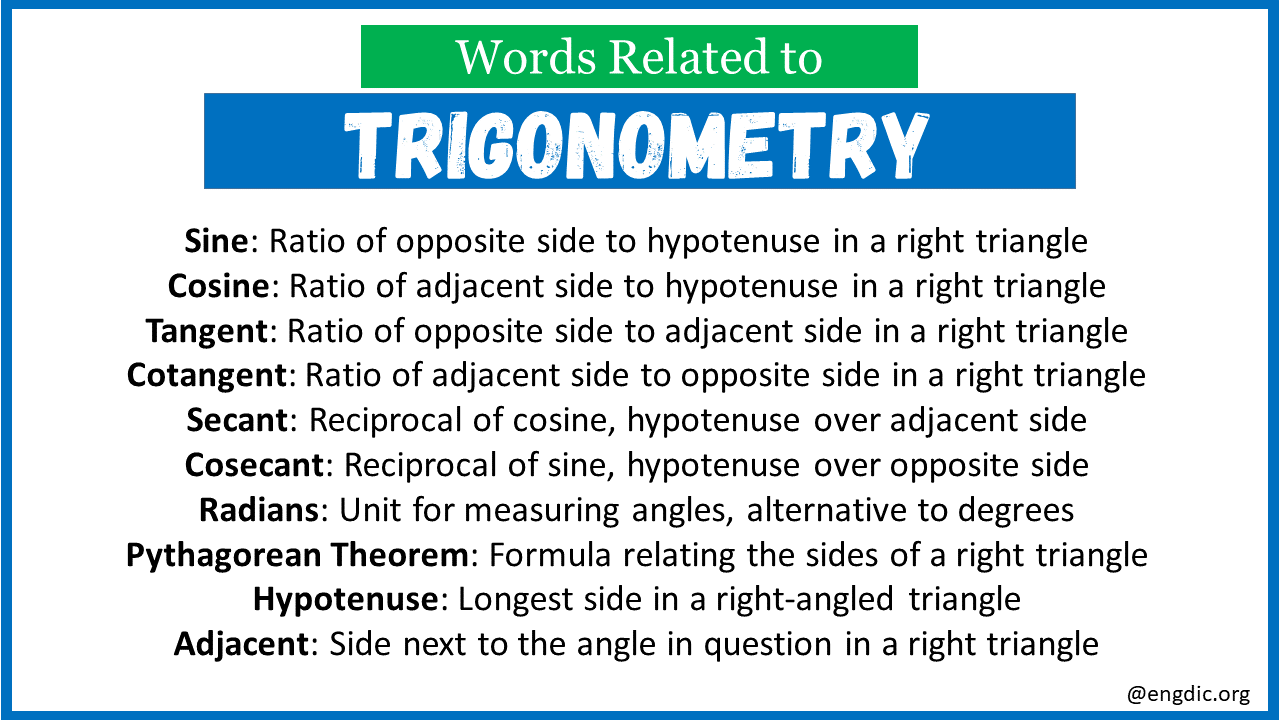# Top 30 Words Related to Trigonometry

Trigonometry is a branch of mathematics that focuses on the relationships between the angles and sides of triangles, especially right-angled triangles. It is widely used in various fields like engineering, physics, and astronomy. Understanding its terminology is crucial for mastering the subject.

## Words Related to Trigonometry

Here are the top 30 terms related to Trigonometry with meanings:

1. Sine: Ratio of the opposite side to the hypotenuse in a right triangle
2. Cosine: Ratio of the adjacent side to the hypotenuse in a right triangle
3. Tangent: Ratio of opposite side to adjacent side in a right triangle
4. Cotangent: Ratio of the adjacent side to the opposite side in a right triangle
5. Secant: Reciprocal of cosine, hypotenuse over adjacent side
6. Cosecant: Reciprocal of sine, hypotenuse over opposite side
7. Radians: Unit for measuring angles, alternative to degrees
8. Pythagorean Theorem: Formula relating the sides of a right triangle
9. Hypotenuse: Longest side in a right-angled triangle
10. Adjacent: Side next to the angle in question in a right triangle
11. Opposite: Side opposite to the angle in question in a right triangle
12. Unit Circle: Circle of radius one used in trigonometry
13. Angle: The space between two intersecting lines or surfaces
14. Arc: Part of a curve, often used in the context of circles
15. Trig Functions: General term for sine, cosine, tangent, etc.
16. Identity: An equation that is true for all its variables
17. Inverse: Function that reverses another function
18. Theta: Greek letter commonly used to represent an angle
19. SOHCAHTOA: Mnemonic for sine, cosine, and tangent formulas
20. Polar Coordinates: System of coordinates using radius and angle
21. Amplitude: Maximum value of a trigonometric function
22. Phase: Horizontal shift of a trigonometric function
23. Cycle: One complete wave of a trigonometric function
24. Frequency: Number of cycles per unit time
25. Asymptote: Line approached by a curve as they head towards infinity
26. Oscillation: Repeated variation in a trigonometric function
27. Periodic: Repeating at regular intervals
28. Quadrant: One of the four regions in a Cartesian coordinate system
29. Pi: Mathematical constant, the ratio of circumference to diameter
30. Chord: Straight line connecting two points on a curve

Explore More Related Words:

Words Related to Trucking

Words Related to Trout

Words Related to Truth